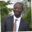RELATED TAGS

python

# How to use the input()function in Python for math operationsAbel Lifaefi Mbula

### Overview

Python is a powerful language. We can use it to build desktop apps, web apps, and much more. In this shot, we'll learn to use the input() function and a basic CLI calculator with Python to do basic math operations like addition, subtraction, multiplication, and division.

To get started, let's try to print the result of an addition of two numbers.

print('Welcome to the CLI calculator')
a = 5
b = 10

print(a + b)

### Explanation

• Lines 1-4: We'll import the CLI calculator. We'll store two numbers in variables a and b.
• Line 6: We'll print the result of the addition.

Instead of hard-coding data, let's take them from the user.

print('Welcome to the CLI calculator')

# Get user input
first_number = input('Add the first number here: ')
second_number = input('Add the first number here: ')

print(first_number + second_number)

We'll use the input() function to get data from the user. The code above won't provide the expected output. Why? See the reason below.

Before discussing the reason why we didn't get the expected result in the code above, let's discuss the input()function. Its syntax is as follows:

input([<prompt>])

This function pauses program execution to allow the user to type in a line of input from the keyboard. Once the user presses the Enter key, all characters typed are read and returned as a string. Because the input values are of type string, not number, 1 + 1 is 11 (concatenation) and not 2 (addition).

The solution is to cast the string to an integer: int().

# Get user input as number
int(input([<prompt>]))

At this point, we are able to do an addition. For the remaining operation, let's add a new variable to hold the type of operation the user wants and use if statements to output the result according to the user's need.

# previous code here...
operator = input('Specify the math operation here (+, -, *, /, %): ')

if(operator == '+'):
print(first_number + second_number)
elif(operator == '-'):
print(first_number - second_number)
elif(operator == '*'):
print(first_number * second_number)
elif(operator == '/'):
print(first_number / second_number)
elif(operator == '%'):
print(first_number % second_number)
else:
print('Sorry, but I cannot understand your operation')

if statements

Note: This code can be improved by using pattern matching (match ... case).

### Conclusion

We'll combine all the data and codes together and we're ready to test our basic calculator with just a basic refactor.

# Calculator
def calculate(first_number, second_number, operator):
if(operator == '+'):
return first_number + second_number
# Substraction (-)
elif(operator == '-'):
return first_number - second_number
# Multiplication (*)
elif(operator == '*'):
return first_number * second_number
# Division (/)
elif(operator == '/'):
return first_number / second_number
# Modulo (%)
elif(operator == '%'):
return first_number % second_number
# Unknown
else:
return 'Sorry, but I cannot understand your operation'

# initialization
def init():
print('Welcome to the CLI Calculator')

first_number = int(input('What\'s the first number? '))
operator = input('Please add the math operator (+, -, *, /, %)? ')
second_number = int(input('What\'s the second number? '))

print(first_number, operator, second_number, ' = ', calculate(first_number, second_number, operator))

# call the initializer
init()


Here, we create two functions. One (calculate()) for the math operation and the other one (init()) for the initialization.

RELATED TAGS

python

CONTRIBUTORAbel Lifaefi Mbula
RELATED COURSES

View all Courses

Keep Exploring

Learn in-demand tech skills in half the time